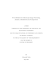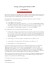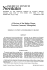# Skeleton-based Hierarchical Shape Segmentation

## Transcription

Skeleton-based Hierarchical Shape Segmentation
```Skeleton-based Hierarchical Shape Segmentation
Dennie Reniers, Alexandru Telea
Department of Mathematics and Computer Science
Eindhoven University of Technology
PO Box 513, 5600 MB Eindhoven, The Netherlands
[email protected], [email protected]
Abstract
We present an effective framework for segmenting 3D
shapes into meaningful components using the curve skeleton. Our algorithm identifies a number of critical points on
the curve skeleton, either fully automatically as the junctions of the curve skeleton, or based on user input. We use
these points to construct a partitioning of the object surface
using geodesics. Because it is based on the curve skeleton, our segmentation intrinsically reflects the shape symmetry and topology. By using geodesics we obtain segments
that have smooth, minimally twisting borders. Finally, we
present a hierarchical segmentation of shapes which reflects
the hierarchical structure of the curve skeleton. We describe
a voxel-based implementation of our method which is robust
and noise resistant, computationally efficient, able to handle shapes of complex topology, and which delivers levelof-detail segmentations. We demonstrate the framework on
various real-world 3D shapes.
1. Introduction
Shape segmentation is the task of decomposing a 3D
shape into its meaningful components. In this context,
meaningful components are those that a human being intuitively perceives as distinct, logical parts of the shape.
Segmentations are useful in shape analysis, shape matching, medical imaging, collision detection, and other geometric processing methods employing divide-and-conquer
strategies.
In defining what characterizes meaningful components,
several directions have been pursued in the past. One of
these is to use curve skeletons. The curve skeleton is a
compact shape descriptor, much like a stick-figure representation. The curve skeleton of a 3D shape is a connected
1D structure that is centered within the shape, reflects its
circular symmetries, and efficiently captures its topological
and geometrical properties . The structure of the curve
skeleton, consisting of branches and junctions, reflects the
hierarchy of the meaningful components. The branches are
associated with the components, whereas the junctions reflect the relationship between components.
In this paper we present a new framework for hierarchical shape segmentation using the curve skeleton. The curve
skeleton is formally defined in terms of geodesics on the
object’s surface . For each curve skeleton point these
geodesics divide the boundary into a set of connected components, providing a natural skeleton-to-boundary mapping
that is intrinsic to the definition of the curve skeleton. Being geodesics, segment borders are smooth and minimally
twisting. After computing the meaningful components, we
combine them into a hierarchical level-of-detail segmentation. Although we use the curve skeleton junctions to provide the meaningful components, other points can be used
as well, depending on the target application. Besides the
segmentation framework, this paper contributes a robust algorithm for computing the curve skeleton of voxelized objects which enhances the method in , and a method to
robustly detect junctions.
Figure 1 provides an overview of our approach, consisting of four stages. First, the curve skeleton is computed
from the voxelized input shape. Second, the critical points
are chosen either automatically as the junctions of the curve
skeleton, or manually by the user. Third, we compute the
component sets of the critical points. Finally, a level-ofdetail segmentation is created from the component sets.
The outline of this paper is as follows. In the next section we review related work. In Section 3 we present some
preliminary definitions. In Section 4 we provide the details of the curve skeleton definition and computation. In
Section 5 we elaborate on the computation of the component sets, and how based on them junctions can be detected
robustly. Section 6 deals with creating the hierarchical segmentation from the component sets. In Section 7 we present
the results of the algorithm. Section 8 presents a discussion
and we conclude in Section 9.
Figure 1. Overview of our segmentation framework.
2. Related Work
Shapes can be segmented by considering their boundary or interior. Approaches that are based on the boundary
usually define segment borders at object-surface concavities. Katz and Tal  use fuzzy clustering on geodesic and
angular distances between surface elements to obtain a hierarchical mesh decomposition with non-jaggy borders. They
show that their segmentation can be used to compute a (control) curve skeleton. In , pose-invariant segmentations
are produced by extracting feature points and cores. In ,
a fuzzy clustering on quasi-flat surface features separated
by curvature extrema is used to obtain a multiscale segmentation of point sets. In , an automatic scissoring scheme
is proposed based on 3D snakes.
Segmentation methods using the curve skeleton consider
the shape’s interior. Our approach falls into this category.
To obtain meaningful components, these methods require
a mapping from the curve skeleton to the object boundary.
Various mappings have been proposed. In  for example,
a combination of planar cross-sections and space sweeping
is used. The approach in  uses force-following of boundary particles onto the curve skeleton. A comparative study
of some of the latest segmentation methods can be found
in .
Our segmentation approach is similar to that of
Li et al. , in which the segmentation is also based on
curve skeletons. However, there are some important differences. Our curve skeleton algorithm has a more fundamental underpinning (see  for details), is more noise resistant, and is connected by default. Li et al. use a planar
cross-section sweeping along the curve skeleton between
critical points to obtain components. The definition of our
curve skeleton is such that it provides a natural skeletonto-boundary mapping. Instead of taking the planar crosssection as borders between components, we use the actual
curve skeleton definition and take the shortest geodesics between feature points as borders. This makes for more natural borders between segments (compare e.g. the Hand object in Fig. 11 with [15, Fig.13]). Furthermore, our framework provides a hierarchical segmentation.
Figure 2. The surface skeleton with rainbow
color map indicating geodesic length (blue is
short, red is long) (a). The curve skeleton C,
a selected point p ∈ C, and Γ(p) = {γ1 , γ2 , γ3 }
(b).
3. Definitions
The surface skeleton S of a 3D object Ω with boundary ∂Ω is defined as those points in Ω having at least two
boundary points at minimum distance:
S ={p ∈ Ω|∃a, b ∈ ∂Ω, a 6= b,
dist(p, a) = dist(p, b) = min dist(p, k)},
(1)
k∈∂Ω
where dist is the Euclidean distance in R3 . Points a, b are
the points at minimum distance of p and are called the feature points of p. Let F : Ω → P(∂Ω) be the feature
transform which assigns to each object point its set of feature points, where P denotes the power set. The surface
skeleton consists of 2D manifolds, called sheets, and of 1D
curves . The sheets intersect in sheet-intersection curves.
Points on these intersection curves have at least three feature
points. The algorithms we present in this paper all work in
binary voxel space . When used in a discrete-space context, Ω denotes the set of foreground, or object voxels.
4. Curve skeleton computation
First, we define the curve skeleton C for the generic
case, namely when the curve skeleton is not (partly) incident with a sheet-intersection curve, so that there are exactly
two feature points for each point p ∈ C. The curve skeleton is defined as those points having at least two shortest
geodesics γi , γj between their two feature points F (p):
p ∈ C ⇔ ∃ two shortest geodesics γi 6= γj between F (p)
(2)
A similar definition was first presented in , and in .
This definition ensures that the curve skeleton is centered
in two ways. First, the curve skeleton is included in the
surface skeleton, as the surface skeleton is defined as those
points having at least two feature points. Second, it is also
centered within the surface skeleton structure, as the two
shortest geodesics γi , γj necessarily have the same length.
In the non-generic case, the curve skeleton is partly incident with a sheet-intersection curve, and there are more than
two feature points for a point p ∈ C. For example, Figure 2a
depicts the surface skeleton of a box with a vertical ridge.
Figure 2b depicts the curve skeleton of the same object.
Point p lies on the sheet-intersection curve of the box’s sheet
and the ridge’s sheet, and has three feature points. Point p
clearly is a curve skeleton point, but definition (2) does not
detect this. Consequently, this definition cannot be used to
detect all curve skeleton points, so we modify it as follows.
With each intersecting sheet a feature-point pair a, b is associated and thus a shortest geodesic γi between a, b. For
example, for three intersecting sheets there are three shortest geodesics (Fig. 2b), not necessarily of the same length.
We call the combination of these shortest geodesics for a
surface skeleton point p ∈ S the shortest-geodesic set Γ(p):
Γ(p) = {γi }i ,
(3)
where γi is a shortest geodesic between the feature-point
pair a, b ∈ F (p) associated with a sheet.
Both in the generic and non-generic case, the union of
shortest geodesics form a Jordan curve on the object surface ∂Ω. Hence, detecting a curve skeleton point p comes
down to detecting whether Γ(p) contains a ring, i.e. computing the genus of Γ(p). By the genus of Γ, we mean the
genus of the surface that is obtained by taking a infinitesimal dilation of Γ on ∂Ω. The new definition of the curve
skeleton replaces (2) and becomes:
p ∈ C ⇔ genus Γ(p) ≥ 1
(4)
Additionally, the genus of Γ can be used to differentiate between junction and non-junction points, called regular
points. A junction is a point on the curve skeleton where at
least three branches come together. The shortest-geodesic
set of such a junction is the union of the Jordan curves of
the neighboring regular points. Hence, if the genus of Γ(p)
is larger than 1, p is a junction:
p ∈ junctions(C) ⇔ genus Γ(p) ≥ 2
(5)
As mentioned, a similar curve skeleton definition was
first presented by Dey and Sun , namely as the set of singular points of the the so-called medial geodesic function,
which assigns to each surface skeleton point the length of
the geodesic between its two feature points, and they show
that this curve skeleton is homotopy equivalent to the original shape. In contrast, we detect the curve skeleton by
performing a topological analysis of the shortest geodesic
set. This definition allows us to compute the curve skeleton without first detecting the surface skeleton, and readily allows junction detection. At an implementation level,
whereas  uses polygonal representations, our implementation of the curve skeleton detection is voxel-based, as detailed in the next section.
4.1. Algorithm
Based on the above definitions, we now present our algorithm for computing the curve skeleton of a voxelized object
Ω. The algorithm consists of several steps that can be executed in parallel for each object voxel. The different stages
are depicted in Figure 3. In the first step, we compute the
feature transform F and extended feature transform F of
Ω. The extended feature transform merges the feature set
of each
S voxel p with that of its first octant 26-neighbors:
F = x,y,z∈{0,1} F (px + x, py + y, pz + z). The result is
that a regular curve skeleton point has two (compact) groups
of feature voxels (Fig. 3a), one on each side of the surface skeleton. The extended feature transform solves two
problems. First, it solves the well-known problem that in
discrete space the feature set of a surface skeleton voxel
might contain only one feature voxel, since there might
be no voxel exactly in the center of the voxelized boundary [6, 18]. Second, it solves the related problem that on a
voxelized object surface, there is typically only one shortest path between the two feature voxels of a curve skeleton
voxel, although there are two on the underlying continuous
surface.
After computing F , we compute the shortest-geodesic
set Γ(p). Because we perform our computations in discrete
voxel space, we compute the shortest geodesics as shortest paths in the boundary graph in which the surface voxels are the nodes and their neighborhood relations represent the edges. The shortest paths are represented as 3D
chain codes  in the boundary graph. For computing a
shortest path we use the A* algorithm , a modification of
Dijkstra’s algorithm, with Euclidean distance as the search
heuristic. We compute a shortest path between each two
feature voxels in F (p). Because F (p) contains groups of
voxels, we will find numerous shortest paths for a regular
point having only two feature points. Together, they form a
discrete (noisy) Jordan curve on the object surface (Fig. 3b).
After computing the path set Γ(p), we determine its
Figure 3. Curve skeleton computation and junction detection.
Figure 4. The boundaries of two dilated pathsets.
genus on ∂Ω, as follows. First we dilate Γ(p) so that we
obtain a surface band Γ0 (p) centered at the 1D structure
Γ(p) (Fig. 3c). Conveniently, small holes from the path set
that are caused by the discrete nature of the shortest paths
(Fig. 3b) are removed by the dilation. The dilation is performed by propagating the voxels in Γ(p) a short distance
outward using a distance-ordered flood fill. Next, we determine the number of compact boundary pieces that the
area Γ0 (p) has. If two or more boundaries are found, p is
concluded to be a curve skeleton voxel (Fig. 3d). When
only one boundary is found, the voxel is concluded to be
a non-curve skeleton voxel (Figure 4a). Empirical studies
on an extensive family of real-world 3D shapes show that a
(geodesic) dilation distance of 5.0 is enough to obtain two
disjoint boundaries if Γ(p) is the discrete representation of
a Jordan curve. In principle, when three or more boundaries
for Γ0 (p) are found, p is a junction. In Figure 4b for example, three boundaries are found for the junction voxel. However, due to the discrete nature of the shortest paths, curve
skeleton voxels might be incorrectly classified as junctions,
i.e., junction detection by analyzing the genus of Γ is conservative. This is problematic if we want to use the junctions in segmentation (Sec. 6). We address this problem in
Section 5.4.
The steps described above (Fig. 3a-d) are executed for all
object voxels in parallel, resulting in the curve skeleton C
(Fig. 3e). Figure 5 shows four curve skeletons as computed
Figure 5. The curve skeleton C of several objects.
by our algorithm. We stress that these results are obtained
without any post-processing, and that our algorithm does
not perform any thinning or erosion step. The curve skeletons, and consequently their junctions, are up to 2 voxels
thick, due to the extended feature transform. We observe
that the curve skeleton is homotopic to the object, and is
connected, although some noise is present. In Section 5.3
we present an importance measure for C that can be used to
obtain noise-resistant, simplified skeletons. This measure is
based on the component sets that are associated with each
point p ∈ C, as explained in the next section.
5. Component Sets
In the previous section we described the detection of a
curve skeleton point p by analyzing the genus of its associated geodesic set Γ(p). In this definition of the curve
skeleton lies a natural skeleton-to-boundary mapping. For
objects of genus 0, the Jordan curve theorem states that the
Figure 7. An object with two tunnels and Γ of
four selected points.
Figure 6. The component sets (bottom) of two
selected points p, q (top).
Jordan curve Γ associated with a regular point divides the
object surface ∂Ω into two connected components. For a
junction point, Γ is the union of Jordan curves of neighboring regular points, dividing the boundary into multiple
components. The component set of a point p is denoted
C(p). Let the k components in C be ordered by their areas:
∀1≤i<k |Ci | ≤ |Ci+1 |. The area of a component Ci (p) is
determined simply by counting its voxels: the cardinality of
the set Ci (p). Although we could use a more sophisticated
surface estimator, the cardinality is sufficient for our purposes. In our voxel space representation, the component set
C(p) for a voxel p is computed by labeling the connected
components in the boundary graph from which the voxels
from the path set Γ(p) are removed. The labeling is sped up
using a simple spatial-subdivision scheme.
Figure 6 shows the component sets for two selected
curve skeleton points p, q, where p and q are a junction and
regular point respectively. The component sets of the junctions are used to obtain a segmentation in Section 6.
5.1. Topological Properties
It is important to note that the number of components
|C(p)| for a point p ∈ C is related to whether the shape Ω
has tunnels, as this will affect the final segmentation. For
shapes without tunnels or holes (genus 0), the curve skeleton has a tree structure. For objects with tunnels (genus ≥
1), the curve skeleton is a graph that contains a loop around
each tunnel. Since there is a skeleton-to-boundary mapping
through the use of Γ, whether Γ(p) divides the boundary
into multiple components is equivalent to whether p divides
the graph into multiple components. In graph theory, a point
p dividing the graph into multiple connected components is
called a cut vertex . The number of components |C(p)|
is related to the genus of Γ(p) and the amount of loops L(p)
that p is on:
|C(p)| = max genus(Γ(p)) + 1 − L(p), 1 .
(6)
Figure 7 shows an example shape with two tunnels and four
selected points. Points p, q are regular (non-junction) points
(genus(Γ(p)) = 1, genus(Γ(q)) = 1), whereas r, s are junctions of three branches (genus(Γ(r)) = 2, genus(Γ(s)) =
2). Point p is not on a loop, points q, r are both on one loop,
and s is on two loops. Indeed, we verify that the cardinality
of the component sets generated by p, q, r, s is 2, 1, 2, 1 respectively. Points p, r are cut vertices in the curve skeleton
graph, whereas q, s are not.
5.2. Inclusion Property
An important property of the component sets for the purpose of hierarchical segmentation is that any two components Ci (p), Cj (q) for two points p, q ∈ C cannot partly
overlap:
Ci (p) ∩ Cj (q) ∈ {∅, Ci (p)}
if |Ci (p)| ≤ |Cj (q)|
(7)
In order to prove (7) for two regular points p, q, we have
to prove that the Jordan curves Γ(p) and Γ(q) cannot intersect. The proof can be roughly sketched as follows. Take
that subset Cpq ⊂ C that is between p and q. It is reasonable to assume that the feature points F (Cpq ) generate two
curves L1 , L2 on ∂Ω, one on each side of the surface skeleton. Each geodesic γ ∈ Γ has one endpoint on L1 and the
other endpoint on L2 . Such a geodesic γ does not intersect
L1 or L2 in any other point as it would no longer be the
shortest geodesic. Now, if a geodesic γp ∈ Γ(p) intersects
γq ∈ Γ(q), it needs to have (a multiple of) two intersection points. But if γp intersects γq in two points a, b, the
shortest geodesic between a, b would not be unique, which
is impossible.
5.3. Importance Measure
The component sets can be used to define an importance
measure for computing simplified curve skeletons that are
robust to noise, as follows. The importance measure ρ(p)
for a point p ∈ C is defined as the importance of p in representing the original shape. One way of expressing ρ(p)
is in terms of the component areas associated with p. Recall that the components in C are ordered by their areas,
or voxel count: ∀1≤i<k |Ci | ≤ |Ci+1 |. We call the largest
component Ck the background component. The others are
called foreground components. The importance measure ρ
for a point p is defined as the total area of the foreground
components:
ρ(p) =
1 [
Ci (p)
|∂Ω|
(8)
1≤i<k
We normalize ρ by the total object surface area |∂Ω|. Note
that this importance measure can only be computed for nonloop points. A regular loop-point has only one component
and no foreground components (e.g. Fig. 7, point q), so that
ρ is undefined. Simplified curve skeletons can be computed
from a noisy curve skeleton C by discarding all points with
an importance below a certain threshold τ :
Figure 8. Conservative junctions J0 (a). Robust junctions Jτ (b).
that are larger than τ :
Fτ (p) =
Cτ = {p ∈ C | ρ(p) ≥ τ }
Jτ =
p ∈ J0 |Fτ (p)| ≥ 2
Ci (p) 1 ≤ i < k ∧
1
|∂Ω| |Ci (p)|
(10)
≥ τ , (11)
(9)
The threshold τ is a user-parameter which controls what is
considered noise. The meaning of this parameter is intuitive: all C-points representing a smaller surface area than
τ are discarded. Furthermore, Cτ can be considered a multiscale representation of the curve skeleton. The simplified
skeletons are connected by default at all scales, because ρ
is monotonic, which follows from (7). The simplified curve
skeletons Cτ of several shapes are shown in Figure 11, with
τ = 10−3 . Thus, curve skeleton points representing an area
less than 0.1% of the object surface are discarded.
This definition of ρ has been presented in . There,
the curve skeleton detection was integrated with the computation of ρ. In contrast, the skeletonization method presented here computes a curve skeleton by analyzing the
genus of Γ, making it much faster to compute, and making it possible to handle objects with tunnels. Furthermore,
in this paper we also present a method to robustly detect
junctions, as detailed in the next section.
5.4. Robust Junction Detection
As indicated in Section 4.1, the genus of Γ(p) is a conservative criterion for detecting junctions, which is problematic if we want to use them for segmentation. Inspired by
the importance measure from the previous section, we discard junctions which have small components among their
foreground components. These junctions are likely the result of noise on the object boundary, or due to discretization
artifacts. A point p is a junction, p ∈ Jτ , at a certain scale τ
if and only if it has at least two foreground components Fτ
where J0 is the set of conservatively detected junctions from
Section 4.1. Although related to the computation of ρ, we
do not have to compute ρ to compute Jτ : We only have to
compute the component sets for the conservative junctions
J0 . Equivalent to the computation of Cτ , the scale τ is used
to distinguish between small-scale noise and signal. For all
objects we tested, a value of τ = 10−3 gives good results.
Like the importance measure, a limitation of this method is
that junctions on C-loops are discarded, as these junctions
have only one foreground component (Sec. 5.1).
In Figure 8a, the conservative junctions J0 on C are
shown for an object with noise on its boundary, computed
by analyzing the genus of Γ. Figure 8b shows the robust
subset selected by applying (10).
6. Segmentation
We now present two methods for computing a shape segmentation from the critical points. By segmentation we
mean the final segmentation S of the object into disjoint
and connected segments Si . In order to obtain a segmentation into meaningful components, the junctions of the curve
skeleton are detected and used as critical points. Alternatively, we can pick critical points by some other (semi) automatic process. The first segmentation method (Sec. 6.1)
we present is the most straightforward. It is based on the
geodesic sets of the critical points, and produces a flat (nonhierarchical) segmentation at the finest scale. The second
method (Sec. 6.2) is based on the component sets generated
by the critical points, and produces a hierarchical, level-ofdetail segmentation.
1:
2:
3:
4:
5:
6:
7:
8:
9:
10:
11:
12:
13:
14:
15:
16:
17:
18:
19:
20:
F ← {Ci (p)|p ∈ P, 1 ≤ i < k} ∪ {C 0 (p)|p ∈ P }
F ← F ∪ {∂Ω}
procedure computeS(τ )
S←∅
for each f ∈ F in ascending order of |f | do
1
|f | ≥ τ then
if |∂Ω|
s←f \S
if f ∩ S 6= ∅ ⇒ |s| > 0.1 · |f ∩ S| then
S ← S ∪ {s}
end if
end if
end for
end procedure
procedure computeLevelsOfDetail()
for each fi , fi+1 in F in ascending order of |f | do
if |fi+1 | − |fi | > 0.1 · |fi | then
1
|fi |)
computeS( |∂Ω|
end if
end for
end procedure
Figure 9. Algorithm for computing a level-ofdetail segmentation.
6.1. Flat Segmentation
We produce a simple, flat segmentation by considering
the shortest-path sets Γ of all critical points P at once.
The connected components in the boundary graph due to
all these shortest paths are labeled. A segmentation that
is obtained in this way is shown in Figure 10b, using the
shortest-path sets of the critical points shown in Fig. 10a.
One property of this segmentation is that it splits tunnelparts of the object into multiple segments.
6.2. Hierarchical Segmentation
In order to produce a hierarchical, level-of-detail segmentation, we consider the component sets C of the critical
points P . In Section 5.3 we distinguished between foreground and background components. In a component set
C consisting of k components C1..k , the largest component
Ck is called the background component, the remaining ones
are called foreground components. The foreground components are those that one would consider meaningful and intuitively associate with the critical point. The background
component is merely the remaining surface area. In Figure 6 for example, the background components are light
gray. Furthermore, we observe that the foreground components combined also form a meaningful component. We denote this compound component by C 0 (p) = ∪1≤i<k Ci (p).
Let F be the set of meaningful components of all critical points combined. The segmentation S should be based
on F , but the components in F are not disjoint. We now
present an algorithm for creating a segmentation S from F
Figure 10. Critical points and their path sets
(a). Flat segmentation (b). Finest level of the
hierarchical segmentation (c).
consisting of disjoint segments Si at a certain scale τ . The
pseudo code of the algorithm is shown in Figure 9.
The idea is that we consider all components f ∈ F in
ascending order of their area (line 5), but only those components that are larger than the specified scale τ (line 6).
The potential segment s is computed as the set difference
between f and the existing segments in S: s = f \ S
(line 7). Before adding the potential segment s to S, we
check whether f overlaps any existing segments from S. If
not, s is added without restriction. If f overlaps, we only
add s if it contributes enough to the segmentation, that is, if
it adds at least 10% of the area that it overlaps (line 8). This
is to prevent tiny segments due to different junctions having
similar components among their component sets. This occurs for example due to the fact that junctions computed by
the algorithm in Section 4 may consist of multiple voxels,
having almost the same component sets. After processing
all components in F , because the background components
have been left out in the segmentation, the object surface
might not be fully covered. Therefore, we add to F the
whole boundary as the largest component (line 2), which
ends up as a single segment filling up the remaining part.
In order to compute multiple segmentations from fine to
coarse (line 14), we can simply consider all components
from F in ascending order of area, and produce a segmentation for each of those areas. To limit the amount of generated hierarchy levels, in our implementation we only compute a hierarchy if two consecutive areas differ by at least
10% from the smaller area fi (line 16). The different segmentations produced at the various scales actually form a
hierarchy, because every segment is included in a segment
from a coarser scale, by (7). Besides being hierarchical, another difference between this segmentation method and the
flat one is that tunnel-parts are not segmented and are left
intact (see Fig. 10c).
7. Results
We have implemented our framework in C++ and have
run it on a Pentium 4, 3GHZ with 1GB of RAM. In computing the shortest paths we used a cache to prevent computing the same shortest path twice. As input we used several
polygonal meshes from [1, 20], voxelized using binvox 
for various resolutions. We used both organic and geometric objects. Figure 11 shows for four objects the simplified curve skeleton Cτ and robust junctions Jτ , both with
τ = 10−3 , and three selected levels from the hierarchical
segmentation. Figure 12 shows for several objects the segmentation at the finest scale. We observe that our method
is able to extract fine details, such as the toes and fingers
of the Armadillo and Dinopet objects, and it does not suffer
from over-segmentation. The use of geodesics as borders of
the surface segments has the very beneficial effect of producing sharp, non-jagged separations, since geodesics are
minimally twisting curves on the surface. Additional results
are available online .
Table 1 shows performance measurements on our framework for several objects that are shown in this paper.
Columns “object”, “dim”, “|Ω|”, “|∂Ω|” denote object
name, voxel-grid dimensions, number of object voxels, and
number of boundary voxels respectively. Column “C time”
denotes the time in seconds to compute the non-simplified
curve skeleton C, including preprocessing (Sec. 4.1). Column “seg time” denotes the time for computing all levels in
the hierarchical segmentation. This time strongly depends
on the amount of levels generated. Column “ρ time“ denotes the time required to compute ρ on C (Sec. 5.3), that is,
to obtain simplified curve skeletons. Note that computing ρ
is not needed for obtaining a segmentation. Column “mem”
gives an indication of the peak memory usage. Computing the curve skeleton takes the most time, which can be
attributed to the computation of the shortest paths and dilations. It takes relatively long for the Plane, which can be
explained by the fact that the Plane has a high surface area
to volume ratio, meaning that the average shortest-path between feature voxels is relatively long. The time needed to
compute the robust junctions Jτ is not shown as it is negligible: up to 5 seconds for the considered objects.
8. Discussion
Following from the curve skeleton definition, the borders of the segments are piecewise geodesic. They do not
the minimal-length path between feature points. Hence, the
borders exhibit minimal twist on the surface, look natural,
and are robust to noise, as shown by the Noisydino object
(Fig. 12). For segmentation methods based on surface curvature, this noise could be problematic .
Segment borders are not based on boundary features but
on the curve skeleton, capturing global geometrical (e.g.
symmetrical) and topological properties. This has a number
of advantages. The segmentations respect the object’s circular symmetry and are invariant for different poses of the
same object. This is because the curve skeleton structure
in general remains stable under deformations of the object.
Figure 13. Pose-invariance
Figure 14. Manual segmentation of the Octopus.
Figure 13 shows the segmentations for two poses of the Cat
(poses from ).
Borders are not necessarily found at curvature extrema.
In the H-shape object for example, borders are found on
flat parts of the surface, segmenting the tips of the H-shape.
These segments are ascribed to the fact that the tips have
sharp convex corners, which generate branches and junctions in the curve skeleton. A consequence of choosing
only the junctions as critical points is that we do not find
segment borders for curvature extrema in tubular-like parts
of the object. In Figure 12 for example, the Dinopet object
has no segment borders on its knees. In order to consider
more the object’s geometry in addition to its topology, we
could choose extra critical points on the curve skeleton at
curvature extrema, computed either on the curve skeleton
or original surface.
As mentioned earlier, our framework supports manual
selection of critical points. In Figure 14a, we manually selected three such points on each tentacle of the Octopus.
The resulting segmentation containing three segments per
tentacle is show in Fig. 14b.
9. Conclusion
We have presented a novel framework for hierarchical
segmentation of 3D shapes. Being based on the formally
defined curve skeleton, our framework has a solid underpinning. The produced segmentations inherit several desirable properties, such as pose-invariance, from the curve
Figure 11. Simplified curve skeletons Cτ , and three levels of the hierarchical segmentation.
Figure 12. Segmentations at the finest scale.
Table 1. Table with measurements. See the text for details.
object
Dinopet
Hand
Horse
Noisydino
Plane
Octopus
dim
188x245x207
334x366x180
366x154x257
366x316x171
125x346x365
217x304x98
366x259x335
|Ω|
905k
1,810k
1,300k
2,038k
1,421k
545k
1,860k
|∂Ω|
80k
136k
94k
119k
114k
110k
154k
skeleton and reflect the symmetry of the object. The definition of the curve skeleton in terms of shortest geodesics
gives rise to a natural skeleton-to-boundary mapping. The
meaningful components are found using the curve skeleton junctions and are combined into a hierarchical, levelof-detail segmentation. Being piecewise geodesic, the segment borders are smooth and non-twisting. Interestingly,
because our method is based on the curve skeleton representing the object’s interior, our method produces both a
surface segmentation and a corresponding volumetric segmentation. The framework supports segmentations based
on the critical points defined as the curve skeleton junctions, but also defined in other ways. A voxel-based implementation is provided. Besides the segmentations, it computes multiscale curve skeletons that are robust to boundary
noise, and performs robust junction detection. We showed
that our framework delivers good results on both organic
and geometric objects. In future work, we would like to detect additional critical points to obtain segment borders at
curvature extrema of the object surface.
Acknowledgement
This work was supported by the Netherlands Organization for Scientific Research (NWO) under grant number
612.065.414.
References
 [email protected]
repository
http://shapes.
aim-at-shape.net.
 M. Attene, S. Katz, M. Mortara, G. Patane, M. Spagnuolo,
and A. Tal. Mesh segmentation - a comparative study. In
Proc. of Shape Modeling International (SMI), 2006.
 U. Clarenz, M. Griebel, M. A. Schweitzer, and A. Telea.
Feature sensitive multiscale editing on surfaces. The Visual
Computer, 20(5):329–343, 2004.
 N. D. Cornea, D. Silver, and P. Min. Curve-skeleton properties, applications and algorithms, 2007. To appear in: IEEE
Trans. on Visualization and Computer Graphics.
 N. D. Cornea, D. Silver, X. Yuan, and R. Balasubramanian.
Computing hierarchical curve-skeletons of 3d objects. The
Visual Computer, 21(11):945–955, 2005.
 L. F. Costa and R. M. Cesar, Jr. Shape analysis and classification. CRC Press, 2001.
C time (s)
42.9
98.3
91.8
105
55.2
228
28.5
seg time (s)
41.0
54.2
20.5
33.7
19.4
20.5
9.47
ρ time (s)
7.50
40.0
5.57
10.2
14.0
38.5
10.5
mem (MB)
447
707
540
660
552
492
600
 T. K. Dey and J. Sun. Defining and computing curveskeletons with medial geodesic function. In Proc. of Eurographics Symposium on Geometry Processing, pages 143–
152, 2006.
 P. Giblin and B. B. Kimia. A formal classification of 3d
medial axis points and their local geometry. IEEE Trans. on
Pattern Analysis and Machine Intelligence, 26(2):238–251,
2004.
 P. E. Hart, N. J. Nilsson, and B. Raphael. A formal basis for
the heuristic determination of minimum cost paths. IEEE
Trans. on Systems Science and Cybernetics, 4(2):100–107,
1968.
 S. Katz, G. Leifman, and A. Tal. Mesh segmentation using feature point and core extraction. The Visual Computer,
21:649–658, 2004.
 S. Katz and A. Tal. Hierarchical mesh decomposition using
fuzzy clustering and cuts. ACM Transactions on Graphics,
22(3):954–961, 2003.
 N. Kiryati and G. Székely. Estimating shortest paths and
minimal distances on digitized three-dimensional surfaces.
Pattern Recognition, 26:1623–1637, 1993.
 T. Y. Kong and A. Rosenfeld. Digital topology: Introduction
and survey. Computer Vision, Graphics, and Image Processing, 48:357–393, 1989.
 Y. Lee, S. Lee, A. Shamir, D. Cohen-Or, and H. P. Seidel.
Mesh scissoring with minima rule and part salience. Computer Aided Geometric Design, 22:444–465, 2005.
 X. Li, T. W. Woon, T. S. Tan, and Z. Huang. Decomposing polygon meshes for interactive applications. In Proc. of
symposium on Interactive 3D graphics, pages 35–42, 2001.
 P. Min. Binvox, a 3d mesh voxelizer. http://www.
 D. Reniers. Personal website, http://www.win.tue.
nl/˜dreniers.
 D. Reniers and A. C. Telea. Quantitative comparison of
tolerance-based feature transforms. In First Int. Conference
on Computer Vision Theory and Applications (VISAPP’06),
pages 107–114, 2006.
 D. Reniers, J. J. Van Wijk, and A. C. Telea. Computing
multiscale curve and surface skeletons of genus 0 shapes using a global importance measure, 2007. Submitted to IEEE
TVCG.
 P. Shilane, P. Min, M. Kazhdan, and T. Funkhouser. The
Princeton shape benchmark. In Shape Modeling International, 2004.
 R. W. Sumner and J. Popovic. Deformation transfer for triangle meshes. ACM Transactions on Graphics, 23(3), 2004.
 D. B. West. Introduction to Graph Theory. Prentice Hall,
1996.
```

### skeleton warriors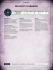### segmentation of cytological images using color and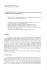### A 3D Live-Wire Segmentation Method for Volume Images Using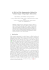### Milestone: Extracting Map Features from Satellite Images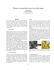### Skeletal histology of Bothriolepis canadensis (Placodermi, Antiarchi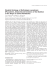### Updated V1.3 of Retired CX TAI/CDI - Rae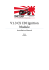### Library-based solutions for algorithms with complex patterns of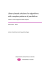### SEGMENTATION OF CYTOLOGICAL IMAGES USING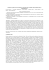### Beşinci Ulusal Deprem Mühendisliği Konferansı, 26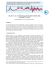### Novel Methods for Microscopic Image Processing